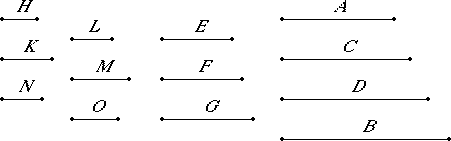# Proposition 21

If two mean proportional numbers fall between two numbers, then the numbers are similar solid numbers.

Let two mean proportional numbers C and D fall between the two numbers A and B.

I say that A and B are similar solid numbers.

Take three numbers E, F, and G, the least of those which have the same ratio with A, C, and D. Then the extremes of them E and G are relatively prime.VIII.20

Now, since one mean proportional number F has fallen between E and G, therefore E and G are similar plane numbers.

Let, then, H and K be the sides of E, and L and M the sides of G.

Therefore it is manifest from the theorem before this that E, F, and G are continuously proportional in the ratio of H to L, and that of K to M.

VII.14

Now, since E, F, and G are the least of the numbers which have the same ratio with A, C, and D, and the multitude of the numbers E, F, and G equals the multitude of the numbers A, C, and D, therefore, ex aequali E is to G as A is to D.

But E and G are relatively prime, primes are also least, and the least measure those which have the same ratio with them the same number of times, the greater the greater and the less the less, that is, the antecedent the antecedent and the consequent the consequent, therefore E measures A the same number of times that G measures D.

Let there be as many units in N as times that E measures A. Then N multiplied by E makes A. But E is the product of H and K, therefore N multiplied by the product of H and K makes A.

Therefore A is solid, and H, K, and N are its sides.

Again, since E, F, and G are the least of the numbers which have the same ratio as C, D, and B, therefore E measures C the same number of times that G measures B.

Let there be as many units in O as times that E measures C. Then G measures B according to the units in O, therefore O multiplied by G makes B.

But G is the product of L and M, therefore O multiplied by the product of L and M makes B. Therefore B is solid, and L, M, and O are its sides. Therefore A and B are solid.

I say that they are also similar.

VII.18

Since N and O multiplied by E make A and C, therefore N is to O as A is to C, that is, E to F.

But E is to F as H is to L, and as K is to M, therefore H is to L as K is to M, and as N is to O.

And H, K, and N are the sides of A, and O, L, and M the sides of B. Therefore A and B are similar solid numbers.

Therefore, if two mean proportional numbers fall between two numbers, then the numbers are similar solid numbers.

Q.E.D.

## Guide

This is a partial converse of VIII.19. It says that if two numbers have two mean proportionals, then they can be viewed as two similar solid numbers. Its proof is analogous the previous proposition dealing with plane numbers, but naturally, it is longer and more involved.

#### Use of this proposition

This proposition is used in VIII.23.